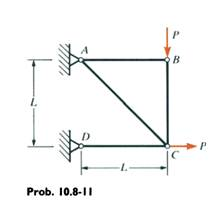### Determine the vertical displacement dc of joint c

Assignment Help Mechanical Engineering
##### Reference no: EM131178202

The truss ABCD shown in the figure is loaded by a vertical force P at joint Band by a horizontal force P at joint C. The axial rigidity of every member is EA. Determine the vertical displacement δc of joint C. (Hint: Add a vertical load Q at joint c.)#### Determine the velocity and acceleration of point

The disk rotates about the shaft S, while the shaft is turning about the z axis at a rate of vz = 4 rad>s, which is increasing at 2 rad>s2. Determine the velocity and accelera

#### Determine the required average blade angle

Determine the required average blade angle at the exit of the impeller if the pump is to deliver 0.57 m3 /s of water with a theoretical head rise of 2.85 m. The rotational s

#### Calculate mean temperature of reception of heat in boiler

Also calculate the mean temperature of reception of heat in the boiler and use this in conjunction with the condenser temperature to evaluate the thermal efficiency. Explain

#### What are the percentages of the total heat absorption

What are the percentages of the total heat absorption taking place in the economizer, boiler drum and the superheater, respectively? Assume cp of air and water as 1.005 kJ/k

#### Driving force for the formation of spheroidite

1. Briefly cite the differences between pearlite, bainite, and spheroidite relative to micro structure and mechanical properties. 2. What is the driving force for the formatio

#### Determine the force in the hydraulic cylinder

Determine the cutting force F applied by the blade DE to the bar at G in terms of the force P applied to the handle of the heavy-duty cutter. Assume that the cutting force F

#### Pressure-angle helical pinion with a right-hand helix

A 17-tooth 20 normal pressure-angle helical pinion with a right-hand helix angle of 30 rotates at 1800 rev/min when transmitting 4 hp to a 52-tooth helical gear. The normal di

#### Determine the maximum length of a cylindrical walnut

It is known that a long log floats with its axis horizontal. A very short log, however, floats with its axis vertical. Determine the maximum length of a cylindrical walnut log

### Write a Review